Math & Science Home | Proficiency Tests | Mathematical Thinking in Physics | Aeronauts 2000

 CONTENTS Introduction Fermi's Piano Tuner Problem How Old is Old? If the Terrestrial Poles were to Melt... Sunlight Exerts Pressure Falling Eastward What if an Asteroid Hit the Earth Using a Jeep to Estimate the Energy in Gasoline How do Police Radars really work? How "Fast" is the Speed of Light? How Long is a Light Year? How Big is a Trillion? "Seeing" the Earth, Moon, and Sun to Scale Of Stars and Drops of Water If I Were to Build a Model of the Cosmos... A Number Trick Designing a High Altitude Balloon Pressure in the Vicinity of a Lunar Astronaut Space Suit due to Outgassing of Coolant Water Calendar Calculations Telling Time by the Stars - Sidereal Time Fields, an Heuristic Approach The Irrationality ofThe Irrationality ofThe Number (i)i Estimating the Temperature of a Flat Plate in Low Earth Orbit Proving that (p)1/n is Irrational when p is a Prime and n>1 The Transcendentality ofIdeal Gases under Constant Volume, Constant Pressure, Constant Temperature and Adiabatic Conditions Maxwell's Equations: The Vector and Scalar Potentials A Possible Scalar Term Describing Energy Density in the Gravitational Field A Proposed Relativistic, Thermodynamic Four-Vector Motivational Argument for the Expression-eix=cosx+isinx Another Motivational Argument for the Expression-eix=cosx+isinx Calculating the Energy from Sunlight over a 12 hour period Calculating the Energy from Sunlight over actual full day Perfect Numbers-A Case Study Gravitation Inside a Uniform Hollow Sphere Further note on Gravitation Inside a Uniform Hollow Sphere Pythagorean Triples Black Holes and Point Set Topology Additional Notes on Black Holes and Point Set Topology Field Equations and Equations of Motion (General Relativity) The observer in modern physics A Note on the Centrifugal and Coriolis Accelerations as Pseudo Accelerations - PDF File On Expansion of the Universe - PDF File

## The Transcendentality ofBy definition, the numberis the ratio of the circumference to the diameter of a circle. This ratio is the same for all circles.is an irrational number. It cannot be represented as the ratio of two integers, regardless of the choice of integers. Equivalently, it cannot be represented as an unending, periodic decimal.is also a transcendental number. It is not a root of any algebraic equation of the form

a0 + a1x + a2x2 + … + anxn = 0

where the ai are all rational numbers and n is finite. For comparison,is also an irrational number. Butis not transcendental since it is a root of the equation

x2 - 2 = 0.

Bothandare irrational, but onlyis transcendental. What makes the difference? One important argument is that a line of lengthcan be constructed using classical techniques (i.e., using compass and straight-edge in a finite number of steps). But, because of the wayis defined, a line of lengthcannot be so constructed. (A curve can. Mark off a unit segment. Bisect the segment. Using the midpoint as center, scribe the appropriate circle. The circle has length.)

To illustrate, let us first consider. To make a construction that produces a line of this length, we begin with two unit-length segments placed end to end so that one segment is at right angles with the other. We then, connect the free ends to complete a right triangle. The new line has lengthNow, consider. A circle of unit diameter has its circumference =. Draw a unit circle, and locate its center. From the center produce a set of n radial lines each separated from its neighbor by an angle 2/n.Approximation for n = 9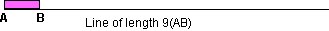Isoseles Triangle

Now, connect the ends with straight line segments to form a set of isosceles triangles. The sum of the lengths of these straight-line segments approaches the circumference of the circle as n approaches infinity (see figure for n = 9).

To construct a line of length, we have but to produce the length AB n times along any line. In the figure, n = 9, AB is a typical straight line segment completing an isosceles triangle, and Circumference9(AB) (see figure).

Now, let us use some algebra to calculate the length AB in the general case. We begin by redrawing part of our previous picture.

One isosceles triangle (ABC with one vertex at the center C and two more vertices on the circle at A and B) is selected (see figure). The angle subtended at C is 2/n. We wish to calculate the length AB, and then to estimate how good an approximation the value n(AB) is to the actual circumference of the circle.

The line CM bisects the angle 2/n, and meets the line AB at right angles. Thus, the triangle CMA is a right triangle, and the length AM = ½(AB).

AM/(Radius) = AM/(½) = sin (/n)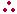AM = ½ sin (/n)

& AB = sin (/n).

Finally, n(AB) = n sin (/n).

Since the actual circumference of the circle is, we now write

n(AB) = {n sin (/n)}/={(sin (/n))/(/n)}

={(sin (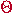))/()}

where=/n.

The value n(AB) differs fromby the multiplicative factor (sin ())/(), with=/n. Notice that, in the limit as n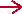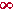,0 and (sin ())/()1. The value n(AB) does indeed approachin the limit. But, while the limit exists, the actual function f() = (sin ())/() does not exist at= 0. It becomes the indeterminate form 0/0. Thus, although n(AB) may approach the actual circumference to any arbitrary precision we might desire, the actual value n(AB) =can never be obtained from any construction of the type outlined above.